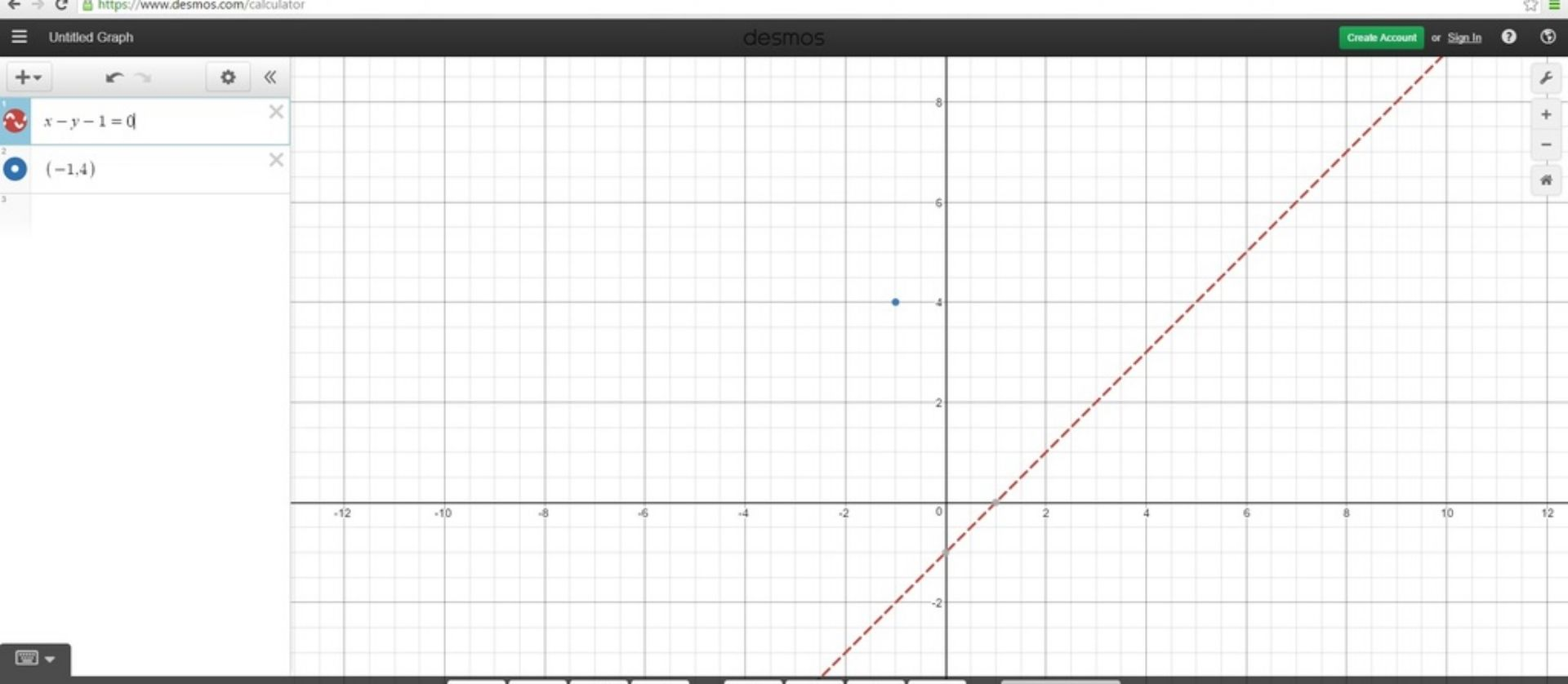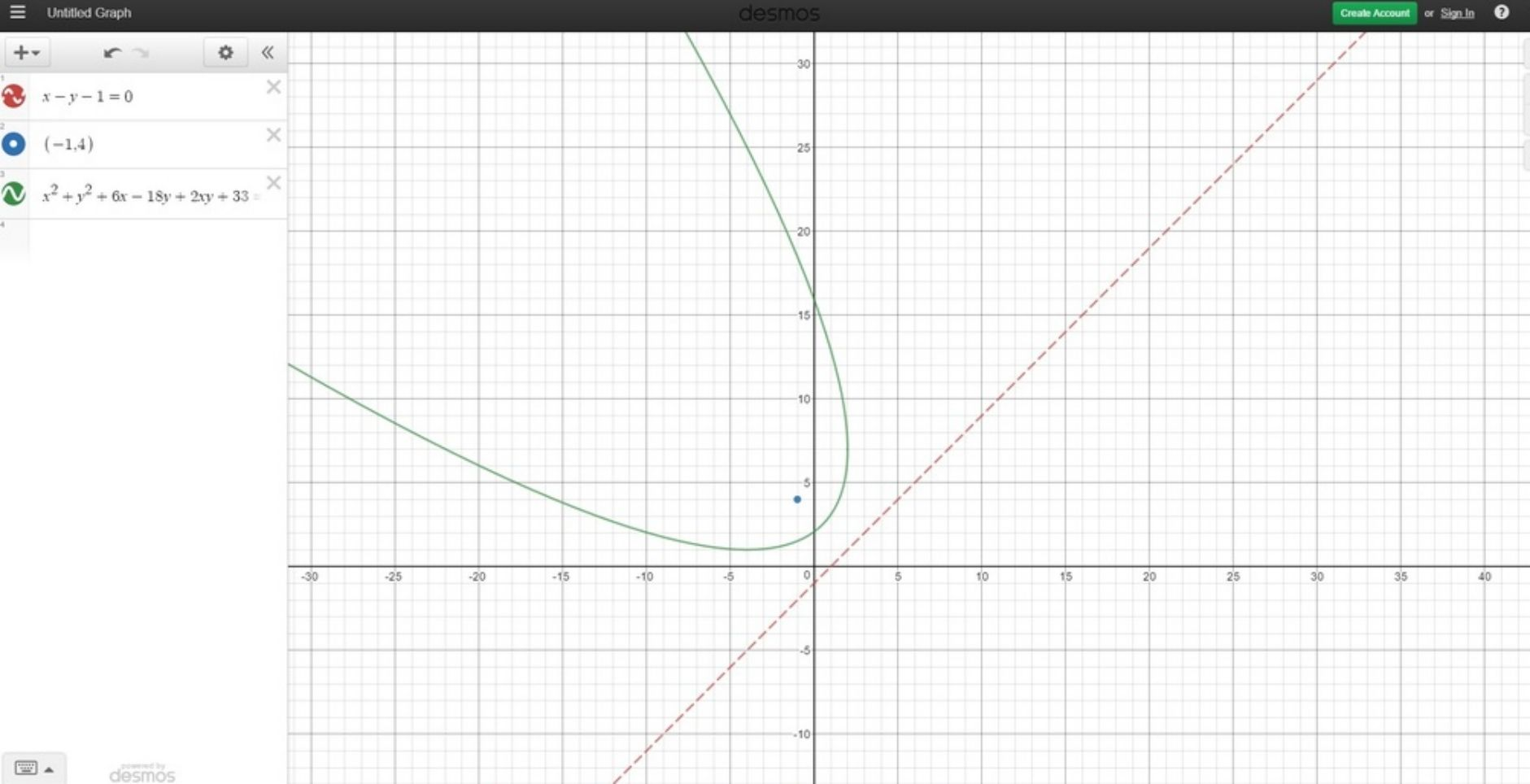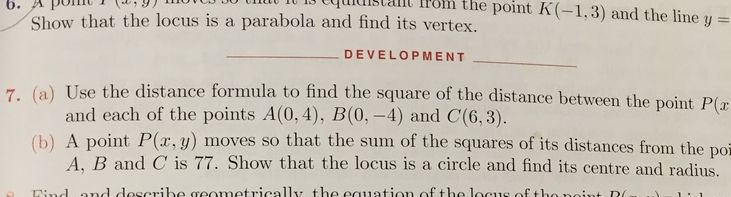# Thread: Cambridge Prelim MX1 Textbook Marathon/Q&A

1. ## Re: Year 11 Mathematics 3 Unit Cambridge Question & Answer Thread

Screen Shot 2016-08-11 at 5.20.50 PM.png

I got c , but the answers says b. Not sure why?

Any one have any clue??Reply With Quote

2. ## Re: Year 11 Mathematics 3 Unit Cambridge Question & Answer ThreadOriginally Posted by appleibeatsScreen Shot 2016-08-11 at 5.20.50 PM.png

I got c , but the answers says b. Not sure why?

Any one have any clue??
From the 'tangent-secant' theorem, we have 10(10+x) = 12^2 = 144, so 10x + 100 = 144, which implies 10x = 44, i.e. x = 4.4, which is option (B).Reply With Quote

3. ## Re: Year 11 Mathematics 3 Unit Cambridge Question & Answer Thread

http://imgur.com/a/3ZNPJ

12a & 13a? (Chapter 9C)Reply With Quote

4. ## Re: Year 11 Mathematics 3 Unit Cambridge Question & Answer ThreadOriginally Posted by pasqhttp://imgur.com/a/3ZNPJ

12a & 13a? (Chapter 9C)
For 12a

The equation of the parabola is in the form

The tangent has the equation

Sub that into the parabola to get a quadratic in terms of x and a, then use the discriminate and let that equal to 0 (since it's a tangent, so there's only one solution) to solve for a. Then sub that value back into the first equation.Reply With Quote

5. ## Re: Year 11 Mathematics 3 Unit Cambridge Question & Answer Thread

Make sure the tangent is effectively portrayedReply With Quote

6. ## Re: Year 11 Mathematics 3 Unit Cambridge Question & Answer ThreadOriginally Posted by integral95For 12a

The equation of the parabola is in the form

The tangent has the equation

Sub that into the parabola to get a quadratic in terms of x and a, then use the discriminate and let that equal to 0 (since it's a tangent, so there's only one solution) to solve for a. Then sub that value back into the first equation.

I haven't checked the answer but would this be right?Reply With Quote

7. ## Re: Year 11 Mathematics 3 Unit Cambridge Question & Answer Thread

Not sure if directrix on the diagonal is covered in Maths Extension 1. I did draw a graph of the points and am aware that the formula from the Focal Point to the straight line is:

Im not sure if the parabola is parallel to the x-axis or parallel to the y-axis.

Here was the diagram I tried to work from :Reply With Quote

8. ## Re: Year 11 Mathematics 3 Unit Cambridge Question & Answer Thread

13a

there might be a shorter wayReply With Quote

9. ## Re: Year 11 Mathematics 3 Unit Cambridge Question & Answer ThreadOriginally Posted by jathu12313a

there might be a shorter way
Think its the first time I have done a question that involved a parabola with a diagonal directrix. (Don't think I have seen too many of those type in past exam papers)

Think I also have to memorise the distance of a point on a parabola to the focus is equivalent to point on a parabola to the directrix. (PS=PM)

When I looked at your final equation, without using a calculator my initial thoughts were it a circle or hyperbola.

But drawing it on desmos it looked like that (not sure how would you describe that graph).Reply With Quote

10. ## Re: Year 11 Mathematics 3 Unit Cambridge Question & Answer Thread

Q 7 (b) EX 9A

I just wondering has there been a mistake in this answer of the 3U Year 11 textbook?

ii) ==> PA² + PB² + PC² = 3x² + 3y² - 14x - 6y + 78 = 77

==> 3x² + 3y² - 14x - 6y = -1

==> x² + y² - 14x/3 - 2y = -1/3

Grouping, (x² - 14x/3) + (y² - 2y) = -1/3

==> (x² - 14x/3 + 49/9) + (y² - 2y + 1) = -1/3 + 49/9 + 1

==> (x - 7/3)² + (y - 1)² = 55/9

The back of the book has the answer as (2,1) for centre and radius ofReply With Quote

11. ## Re: Year 11 Mathematics 3 Unit Cambridge Question & Answer ThreadOriginally Posted by davidgoes4wceQ 7 (b) EX 9A

I just wondering has there been a mistake in this answer of the 3U Year 11 textbook?

ii) ==> PA² + PB² + PC² = 3x² + 3y² - 14x - 6y + 78 = 77

==> 3x² + 3y² - 14x - 6y = -1

==> x² + y² - 14x/3 - 2y = -1/3

Grouping, (x² - 14x/3) + (y² - 2y) = -1/3

==> (x² - 14x/3 + 49/9) + (y² - 2y + 1) = -1/3 + 49/9 + 1

==> (x - 7/3)² + (y - 1)² = 55/9

The back of the book has the answer as (2,1) for centre and radius of
You didn't give us point A B and CReply With Quote

12. ## Re: Year 11 Mathematics 3 Unit Cambridge Question & Answer ThreadOriginally Posted by davidgoes4wceQ 7 (b) EX 9A

I just wondering has there been a mistake in this answer of the 3U Year 11 textbook?

ii) ==> PA² + PB² + PC² = 3x² + 3y² - 14x - 6y + 78 = 77

==> 3x² + 3y² - 14x - 6y = -1

==> x² + y² - 14x/3 - 2y = -1/3

Grouping, (x² - 14x/3) + (y² - 2y) = -1/3

==> (x² - 14x/3 + 49/9) + (y² - 2y + 1) = -1/3 + 49/9 + 1

==> (x - 7/3)² + (y - 1)² = 55/9

The back of the book has the answer as (2,1) for centre and radius of
You made a small silly mistake, the point B has coordinates (0,-4). So PB^2 would be x^2+(y+4)^2Reply With Quote

13. ## Re: Year 11 Mathematics 3 Unit Cambridge Question & Answer ThreadOriginally Posted by pikachu975You didn't give us point A B and C
That was the question: (apologies about the cut off of page)Reply With Quote

14. ## Re: Year 11 Mathematics 3 Unit Cambridge Question & Answer Thread

OK I decided to redo Q 7 a and 7b from EX 9AReply With Quote

15. ## Re: Year 11 Mathematics 3 Unit Cambridge Question & Answer ThreadOriginally Posted by davidgoes4wceYeah your PB is wrong.Reply With Quote

16. ## Re: Year 11 Mathematics 3 Unit Cambridge Question & Answer Thread

Hi, need help with Exercise 8H Page 311 Extension Question 27.
If alpha and beta are the roots of x^2 = 5x - 8. Find (a)^1/3 + (b)^1/3 without finding the roots.
Tried cubing (a^1/3 + b^1/3) but got stuck.
Any help would be appreciated.

Also, with Extension question 26 (same page),
If the equations mx^2 + 2x +1 =0 and x^2 + 2x +m = 0 have a common root, find the possible values of m and the value of the common root in each case. I let first equation be p(x) and the second be f(x) and the common root be alpha.
If alpha is the common root p(a) = f(a) = 0 and hence you get,
a^2(m-1) + (1-m) = 0, and that the common root a is x=-1 when m = 1
I had no idea how to get the second common root of the two equations (its x = 1 when m = -3),
So I used product of roots of the above equation a^2(m-1) + (1-m) = 0 as a quadratic is a, and the product of roots is thus -1
Hence I found the other root to be x = -1 and subbing x = -1 into any of the given functions p(x), f(x) and letting it equal 0 I then got m = -3.
Is there a way to find the 2nd m value prior to finding the 2nd common root?
(because this is what the question seems to imply find m then find the common root)
Sorry for long post couldn't find anything on either of the questions
Thanks!Reply With Quote

17. ## Re: Year 11 Mathematics 3 Unit Cambridge Question & Answer ThreadOriginally Posted by trea99Hi, need help with Exercise 8H Page 311 Extension Question 27.
If alpha and beta are the roots of x^2 = 5x - 8. Find (a)^1/3 + (b)^1/3 without finding the roots.
Tried cubing (a^1/3 + b^1/3) but got stuck.
Any help would be appreciated.

Ok - to save time I will use a and b for alpha and beta.Reply With Quote

18. ## Re: Year 11 Mathematics 3 Unit Cambridge Question & Answer Thread

Yes, I got that part lol.
But what would you do to get a^(1/3) + b^(1/3) from a+b = 5 and ab = 8
What I tried was,
(a^(1/3) + b^(1/3))^3 and got a + 3a^(2/3)b^(1/3) + 3a^(1/3)b^(1/3) + b
Tried doing something with the 3a^(2/3)b^(1/3) + 3a^(1/3)b^(2/3) but ended up getting more a^(1/3) + b^(1/3) terms when I tried factorising by taking out powers of ab
Or would cubing a^(1/3) + b^(1/3) be useless for this question?Reply With Quote

19. ## Re: Year 11 Mathematics 3 Unit Cambridge Question & Answer Thread

Thank you so muchReply With Quote

20. ## Re: Year 11 Mathematics 3 Unit Cambridge Question & Answer ThreadOriginally Posted by trea99Thank you so muchYou were so close to finishing. Never done this type of question before.Reply With Quote

21. ## Re: Year 11 Mathematics 3 Unit Cambridge Question & Answer Thread

EX7D Q 9 (b) is there a mistake with the answer?Reply With Quote

22. ## Re: Year 11 Mathematics 3 Unit Cambridge Question & Answer Thread

One of the angles I believe to be right (the 71 34') and the other I believe to be wrong. Would love it , if somebody could confirm.

Seeing that the two points of intersection are at x=2, -3

I then substituted this back into the derivative which is dy/dx=2x-1=tanReply With Quote

23. ## Re: Year 11 Mathematics 3 Unit Cambridge Question & Answer Thread

The 2 angles in degrees: 71.565 and 98.13

being: inverse tan (3) and 180 - inverse tan (7)

Maybe my calculator is exceptionally accurate.Reply With Quote

24. ## Re: Year 11 Mathematics 3 Unit Cambridge Question & Answer Thread

I had an attempt at the question and I can't quite get itReply With Quote

25. ## Re: Year 11 Mathematics 3 Unit Cambridge Question & Answer Thread

My reasoning as to why I subtract 180 from 81'52' is that the tan theta value is a value of negative 7, which lies somewhere in the 2nd quadrant of the ASTC diagram.Reply With Quote

#### Thread Information

##### Users Browsing this Thread

There are currently 1 users browsing this thread. (0 members and 1 guests)

####Posting Permissions

• You may not post new threads
• You may not post replies
• You may not post attachments
• You may not edit your posts
•